flat assembler
Message board for the users of flat assembler.Index > Main > Compute 10^n/10 using only 1 register with 2 instructions Goto page Previous  1, 2, 3  Next
Author
 Threadrevolution When all else fails, read the source Joined: 24 Aug 2004 Posts: 17457 Location: In your JS exploiting you and your system revolution Oh, we need to do division in the ring. So for 7 we do (6/4) mod 7 = 5 Becomes 2^32 * (6/(2^(32 mod period)) mod 7) / 7 = 0xB6DB6DB728 Jan 2019, 09:46
 Tomasz Grysztar Joined: 16 Jun 2003 Posts: 7750 Location: Kraków, Poland Tomasz Grysztar I do not really understand what you are trying to do here. The construction I've shown does not use division anywhere. For 7 it is all straightforward, because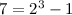, so we already have a "nice" denominator and representation has period of 3. When numerator is -a and a<7, the block of 3 digits that is repeated is the same as binary representation of a: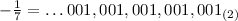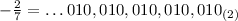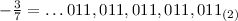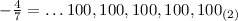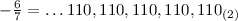If you only need low 32 digits, you just cut it off there (it does not matter that you cut in a middle of a block). For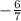you get 0B6DB6DB6h. And you can get the low 32 digits ofby adding 1 to 0B6DB6DB6h. The low 32 digits are the same no matter if you add 1 to a whole 2-adic number or if just to a truncated version.28 Jan 2019, 10:06
 Tomasz Grysztar Joined: 16 Jun 2003 Posts: 7750 Location: Kraków, Poland Tomasz Grysztar In other words: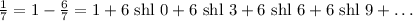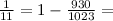You could compute their truncated forms this way directly on a 32-bit CPU: Code:; compute 1/11: mov eax,1 xor cl,cl periodic: mov edx,930 shl edx,cl add eax,edx add cl,10 cmp cl,32 jb periodic  The only hard part, if you wanted to compute 2-adic remainders at run-time, would be to find a period (preferably the minimum one). This means finding such a multiply of denominator that it is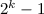for some k. You could apply methods discussed with Chinese remainder theorem. However, this might easily overflow on 32-bit architecture. You may try to design a different algorithm computing 2-adic representation, but please note that 2-adic division is really something different from division of integers or standard base 2 floating-point numbers, even if the patterns of digits appear to be very similar. Numbers in base 2 may have infinite expansions to the right (negative powers of 2), while 2-adic numbers can expand infinitely to the left and the number of digits to the right of the point is always finite. With p-adic arithmetic you have an exact precision on the right side. For example, consider a base 2 representation of, which is 0.01010101010101... If you try to multiply this by 3, the approximations are going to look like 0.1111... - their initial digit is wrong and there is a loss of precision. On the other hand, the 2-adic representation ofis ...0101011. Now if you multiply it by 3, you get ...0001. You can stop at any point, dropping the higher digits, but the ones you have on the right end are already precise and final. This is because the low n digits of the result depend only on low n digits of the factors, so as long as you know the low digits of the factors precisely, you also know the low digits of the product precisely.28 Jan 2019, 10:26
 revolution When all else fails, read the source Joined: 24 Aug 2004 Posts: 17457 Location: In your JS exploiting you and your system revolution I wanted to use the native division instruction. Code:mov ebx,7 ;the divisor lea edx,[ebx-1] ;numerator high xor eax,eax ;numerator low div ebx ;6/7 bad result inc eax ;+1 But of course that is not the correct result. We need to adjust the numerator. Code:mov ebx,7 ;the divisor lea edx,[ebx-1] ;numerator high xor eax,eax ;numerator low ;code to compute (6 shr 2) mod 7 giving edx = 5 div ebx ;5/7 the correct result inc eax ;+1 The modulo division is easy, and I can understand it. The hard part, like you say, is to find the period. I still need to figure out that. Edit: Something like this can do the modulo part Code:PERIOD = 3 mov ecx,32 mod PERIOD .modulo_division: bt edx,0 sbb eax,eax and eax,ebx add edx,eax shr edx,1 loop .modulo_division It just remains to compute the PERIOD value.28 Jan 2019, 11:00
 Tomasz Grysztar Joined: 16 Jun 2003 Posts: 7750 Location: Kraków, Poland Tomasz Grysztar OK, I think I understand now. Basically, to find the low digits ofyou want to compute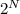divided by 7 (with a regular integer division with remainder), where N is at least 32 and a multiple of the period (3 in this case). Then, because N is a multiple of period: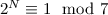and your division gives: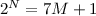. Dividing both sides by 7, we get: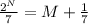And thus:This number only differs from actual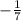on digits starting from position N. Since N is at least 32, it means that low 32 digits of this number are identical to. Now to get low digits ofyou need to multiply this by 6 and then add 1, or alternatively just negate the number (subtract it from 0). Yes, this seems like a valid method of obtaining the low digits of 2-adic fraction, but when N becomes too large it becomes problematic. And, of course, you need to know the period here. I believe there should exist an actual algorithm for p-adic division digit after digit, starting from the lowest one - with such one we would not have to know the period in advance.28 Jan 2019, 11:25
 revolution When all else fails, read the source Joined: 24 Aug 2004 Posts: 17457 Location: In your JS exploiting you and your system revolution The period for 1/37 is 36. I think that can't be done in 32-bit code.28 Jan 2019, 11:28
 Tomasz Grysztar Joined: 16 Jun 2003 Posts: 7750 Location: Kraków, Poland Tomasz Grysztar revolution wrote:The period for 1/37 is 36. I think that can't be done in 32-bit code. Well, you can do it like this: Code:; compute low 32 digits of -1/37: mov edx,1 shl (36-32) xor eax,eax sub eax,1 sbb edx,0 mov ecx,37 div ecx test edx,edx jnz assertion_failed ; negate to get low 32 digits of 1/37: neg eax For very large periods you would need to chain DIV instructions.28 Jan 2019, 11:40
 Tomasz Grysztar Joined: 16 Jun 2003 Posts: 7750 Location: Kraków, Poland Tomasz Grysztar I patched up a code that uses chained DIVs and should work for any odd divisor: Code: mov ecx,2 ; number of dwords, except the highest one all are 0FFFFFFFFh mov ebx,1 ; start with 2^33-1 search: push ecx xor edx,edx mov eax,ebx jmp first_division divide: or eax,0FFFFFFFFh first_division: div [divisor] loop divide pop ecx test edx,edx jz found stc rcl ebx,1 jnc search mov ebx,1 inc ecx jmp search found: ; eax is now low 32 digits of -1/[divisor] neg eax ; negate to get low 32 digits of 1/[divisor]  Last edited by Tomasz Grysztar on 28 Jan 2019, 12:43; edited 1 time in total28 Jan 2019, 11:59
 revolution When all else fails, read the source Joined: 24 Aug 2004 Posts: 17457 Location: In your JS exploiting you and your system revolution Yes, for very large periods I think using a normal native divider is the way. So the code needs to split in two paths, one for short periods where we can use a single imul, and another for other values where we use the div.28 Jan 2019, 12:01
 Tomasz Grysztar Joined: 16 Jun 2003 Posts: 7750 Location: Kraków, Poland Tomasz Grysztar revolution wrote:Yes, for very large periods I think using a normal native divider is the way. So the code needs to split in two paths, one for short periods where we can use a single imul, and another for other values where we use the div. When divisor is an odd number, once you have low 32 bits of its reciprocal, you always use just a single IMUL: Code:; assuming EAX is divisible by 137, compute the quotient: imul eax,077975B9h ; multiply by low 32 digits of 2-adic 1/137 (for 137 the period is 68).28 Jan 2019, 12:05
 revolution When all else fails, read the source Joined: 24 Aug 2004 Posts: 17457 Location: In your JS exploiting you and your system revolution Tomasz Grysztar wrote:When divisor is an odd number, once you have low 32 bits of its reciprocal, you always use just a single IMUL. Okay. I haven't tested it yet, I had just assumed it wouldn't be valid. Good, I'm glad my assumption was wrong.28 Jan 2019, 12:13
 Tomasz Grysztar Joined: 16 Jun 2003 Posts: 7750 Location: Kraków, Poland Tomasz Grysztar revolution wrote: Tomasz Grysztar wrote:When divisor is an odd number, once you have low 32 bits of its reciprocal, you always use just a single IMUL. Okay. I haven't tested it yet, I had just assumed it wouldn't be valid. Good, I'm glad my assumption was wrong.My explanation might have been concise, but if you follow all the details, it shows that all is predicable and precise. As long as the divisor is odd and the number is exactly divisible by it, if you multiply by the low bits of reciprocal, the same number of bits of result are going to be exact, with no exceptions. The only hard part is to find the reciprocal. The algorithms we have here are not efficient. I may think about finding something better. On the other hand, when you know the divisor in advance (which is usual case for replacing division with multiplication), you can take some time to find its 2-adic reciprocal and then just use it.28 Jan 2019, 12:22
 Tomasz Grysztar Joined: 16 Jun 2003 Posts: 7750 Location: Kraków, Poland Tomasz Grysztar All right, I designed much more efficient algorithm for computing 2-adic division. Just put A=1 to compute a reciprocal: Code:divide_2adic: ; in: ; edx = low 32 bits of A ; ebx = low 32 bits of B (must be odd) ; on success: ; cf = 0 ; eax = low 32 bits of 2-adic A/B test ebx,1 jz .invalid xor eax,eax .iteration: mov ecx,eax imul ecx,ebx xor ecx,edx bsf ecx,ecx jz .done bts eax,ecx jmp .iteration .done: clc ret .invalid: stc ret  Code:; a few tests: mov edx,1 mov ebx,3 call divide_2adic ; result: 0xAAAAAAAB mov edx,-1 mov ebx,3 call divide_2adic ; result: 0x55555555 mov edx,1 mov ebx,5 call divide_2adic ; result: 0xCCCCCCCD mov edx,1 mov ebx,7 call divide_2adic ; result: 0xB6DB6DB7 mov edx,1 mov ebx,37 call divide_2adic ; result: 0x914C1BAD mov edx,1 mov ebx,137 call divide_2adic ; result: 0x077975B928 Jan 2019, 13:20
 revolution When all else fails, read the source Joined: 24 Aug 2004 Posts: 17457 Location: In your JS exploiting you and your system revolution I did some more tests. In fact I brute forced the entire 32-bit space. Didn't take too long either, just a few minutes. Using a normal div instead of imul would have taken much longer. Code:format ELF executable 0 entry main SYS_EXIT = 1 SYS_WRITE = 4 STD_OUTPUT = 1 segment executable main: mov esi,1 .loop_divisor: ;limit the print output to avoid spamming the console mov edx,esi and edx,not 1 bsf eax,edx bsr edx,edx sub edx,eax cmp edx,4 - 1 ;if we have no more than the top four bits set then print something ja .done_print mov edx,esi mov ecx,trial.at call write_hex mov ecx,trial mov edx,trial.len call out_message .done_print: ;divide_2adic: ;see post from Tomasz Grysztar at https://board.flatassembler.net/topic.php?p=208280#208280 xor edi,edi .divide_2adic_test: mov ecx,edi imul ecx,esi xor ecx,1 ;fixed numerator of 1 bsf ecx,ecx jz .divide_2adic_done bts edi,ecx jmp .divide_2adic_test .divide_2adic_done: ;edi = the 2-adic divisor xor eax,eax .loop_trials: mov ecx,eax imul ecx,edi imul ecx,esi cmp eax,ecx jne .failed add eax,esi ;only trial proper multiples jnc .loop_trials add esi,2 ;only trial odd numbers jnc .loop_divisor mov ecx,success mov edx,success.len call out_message .close: mov eax,SYS_EXIT xor ebx,ebx int 0x80 .failed: mov edx,esi mov ecx,fail.at call write_hex mov ecx,fail mov edx,fail.len call out_message jmp .close out_message: mov eax,SYS_WRITE mov ebx,STD_OUTPUT int 0x80 retn write_hex: ;ecx = address ;edx = value .next_nibble: mov eax,edx shr eax,28 cmp al,10 sbb al,0x69 das mov [ecx],al inc ecx shl edx,4 jnz .next_nibble retn segment readable writeable trial: db 'Trialing 0x' trial.at db '00000000',10 trial.len = $- trial fail: db 'Failed at 0x' fail.at db '00000000',10 fail.len =$ - fail success: db 'All values passed okay',10 success.len = \$ - success  Quote:All values passed okay Very good28 Jan 2019, 14:13
 Tomasz Grysztar Joined: 16 Jun 2003 Posts: 7750 Location: Kraków, Poland Tomasz Grysztar This also seems like a good place to try out a BMI instruction: Code:divide_2adic: ; in: ; edx = low 32 bits of A ; ebx = low 32 bits of B (must be odd) ; on success: ; cf = 0 ; eax = low 32 bits of 2-adic A/B test ebx,1 jz .invalid xor eax,eax .iteration: mov ecx,eax imul ecx,ebx xor ecx,edx jz .done blsi ecx,ecx or eax,ecx jmp .iteration .done: clc ret .invalid: stc ret28 Jan 2019, 15:22
 revolution When all else fails, read the source Joined: 24 Aug 2004 Posts: 17457 Location: In your JS exploiting you and your system revolution Tomasz Grysztar wrote:This also seems like a good place to try out a BMI instruction: Hmm, I'll try maybe another time. Quote:Illegal instruction (core dumped)28 Jan 2019, 15:38
 revolution When all else fails, read the source Joined: 24 Aug 2004 Posts: 17457 Location: In your JS exploiting you and your system revolution We can also get other ratios with this. For example for division by 5: To get a ratio of 0.2 (1/5) for numbers of the form 5*n use the value 0xcccccccd To get a ratio of 0.4 (2/5) for numbers of the form 5*n use the value 0x9999999a To get a ratio of 0.6 (3/5) for numbers of the form 5*n use the value 0x66666667 To get a ratio of 0.8 (4/5) for numbers of the form 5*n use the value 0x3333333428 Jan 2019, 16:47
 Tomasz Grysztar Joined: 16 Jun 2003 Posts: 7750 Location: Kraków, Poland Tomasz Grysztar Wait, even IMUL is not really needed there. The product can be accumulated instead of being recomputed every iteration: Code:divide_2adic: ; in: ; edx = low 32 bits of A ; ebx = low 32 bits of B (must be odd) ; on success: ; cf = 0 ; eax = low 32 bits of 2-adic A/B test ebx,1 jz .invalid xor eax,eax xor esi,esi .iteration: mov ecx,esi xor ecx,edx jz .done bsf ecx,ecx bts eax,ecx mov edi,ebx shl edi,cl add esi,edi jmp .iteration .done: clc ret .invalid: stc ret28 Jan 2019, 22:30
 revolution When all else fails, read the source Joined: 24 Aug 2004 Posts: 17457 Location: In your JS exploiting you and your system revolution Tomasz Grysztar wrote:Wait, even IMUL is not really needed there. The product can be accumulated instead of being recomputed every iteration: Still passes all test values, and it's slightly quicker on my machine in the full 32-bit range tester. Surprisingly the runtimes are about evenly split between the 2-adic computation and the range trialling checker. But in the final application the 2-adic computation time will be insignificant, which is why I didn't really care about using div. Just that using div was tricky with the period determination.29 Jan 2019, 06:06
 Tomasz Grysztar Joined: 16 Jun 2003 Posts: 7750 Location: Kraków, Poland Tomasz Grysztar You could also use this routine directly, to divide any two numbers. Well, except that B needs to be odd, but if it's even you can start with shifting both A and B right in parallel until B becomes odd: Code:divide_2adic: ; in: ; edx = low 32 bits of A ; ebx = low 32 bits of B ; on success: ; cf = 0 ; eax = low 32 bits of 2-adic A/B ; on failure: ; cf = 1 test ebx,1 jz .shift xor eax,eax xor esi,esi .iteration: mov ecx,esi xor ecx,edx jz .done bsf ecx,ecx bts eax,ecx mov edi,ebx shl edi,cl add esi,edi jmp .iteration .done: clc ret .shift: shr ebx,1 jz .invalid ; divide by 0 shr edx,1 jnc divide_2adic ; underflow .invalid: stc ret  In fact, with this function you get access to all basic arithmetic operations on 2-adic numbers (addition, subtraction, multiplication and division), as long as you can go by having just low 32 digits of the result. ADD, SUB and IMUL work just as well for truncated 2-adic numbers as for integers, so the division is the only operation that needs a specialized routine. What is especially nice about truncated 2-adic arithmetics is that you can use it to perform several operations on rational numbers, and as long as you know that the final result is going to be an integer in 32-bit range, you know that result is going to be precise. You need such additional knowledge because just by looking at the lowest 32 bits of 2-adic result you are not able to tell an integer from a fraction. Within this truncated 32-bit section, arithmetic operations do not lose precision at any point, except when you divide by 2, which is a shift right and can lose a set bit by shifting it out of register (it is a failure condition in the above function). But the number of bits that are shifted into negative range is always finite, so you can get around the problem by shifting your numbers left far enough (and then shift the final result back right). Other than that, you can accumulate as many arithmetic operations on rational numbers as you wish and the result is still going to be exact, no matter how many additions/multiplications/divisions you do: Code:; compute (1/13+8/17)*(210/11+1) = 11 mov edx,1 mov ebx,13 call divide_2adic jc failed push eax mov edx,8 mov ebx,17 call divide_2adic jc failed pop ebx add eax,ebx push eax mov edx,210 mov ebx,11 call divide_2adic jc failed inc eax pop ebx imul eax,ebx cmp eax,11 jne failed29 Jan 2019, 09:30
 Display posts from previous: All Posts1 Day7 Days2 Weeks1 Month3 Months6 Months1 Year Oldest FirstNewest FirstJump to: Select a forum Official----------------AssemblyPeripheria General----------------MainDOSWindowsLinuxUnixMenuetOS Specific----------------MacroinstructionsCompiler InternalsIDE DevelopmentOS ConstructionNon-x86 architecturesHigh Level LanguagesProgramming Language DesignProjects and IdeasExamples and Tutorials Other----------------FeedbackHeapTest Area
Goto page Previous  1, 2, 3  Next

Forum Rules:
 You cannot post new topics in this forumYou cannot reply to topics in this forumYou cannot edit your posts in this forumYou cannot delete your posts in this forumYou cannot vote in polls in this forumYou cannot attach files in this forumYou can download files in this forum